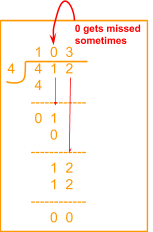In the verge of coronavirus pandemic, we are providing FREE access to our entire Online Curriculum to ensure Learning Doesn't STOP!

# Division

Go back to  'Multiplication and Division'

It is in Grade 2 that students get introduced to the idea of division. Division is best introduced through the use of everyday items and scenarios.

Activities are the best way to begin with eventually moving on to the visual models and finally to abstract numbers.

## Understanding division using objects.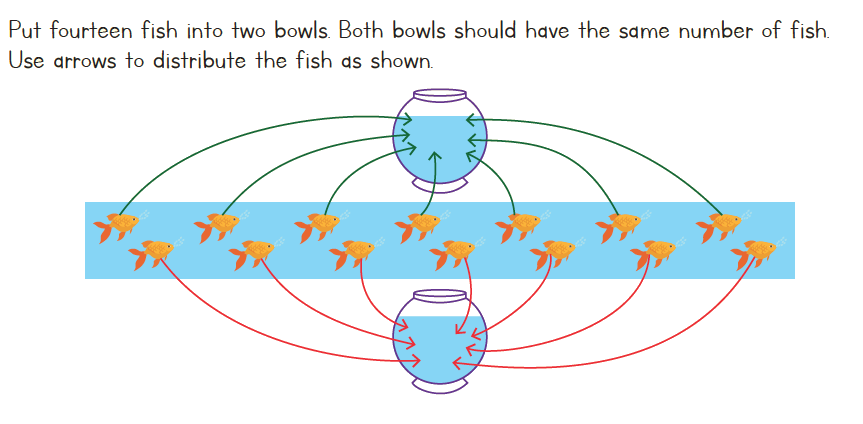As you can see above there will be $$7$$ fish in each bowl. The fishes have been divided equally into $$2$$ bowls. The grouping of objects equally into groups is known as division.

The division statement for the picture above can be written as $$12 \div 3 = 4.$$

Here is another way to understand the grouping of objects, this time using the dots. The example below explains how division statements can be formed based on the number of objects, number of groups and the total number of objects.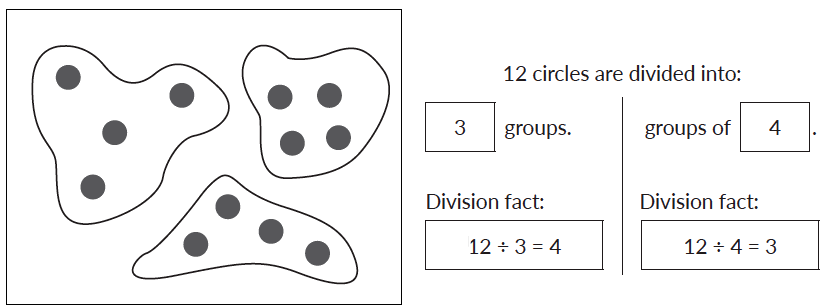## Understanding division using number line

Before moving on to understanding the procedure of long division, let’s try and understand it on the number line. The image given below shows division on a number line.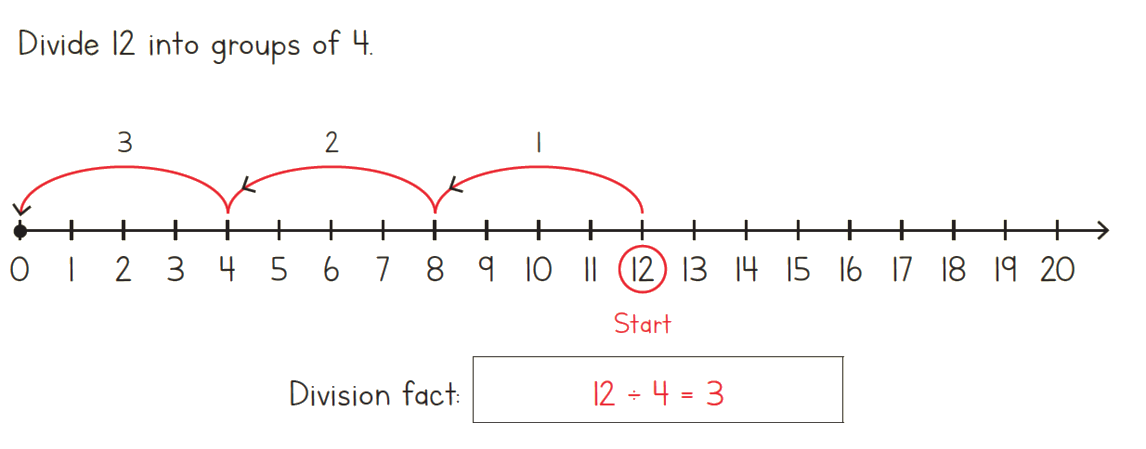## Understanding the terms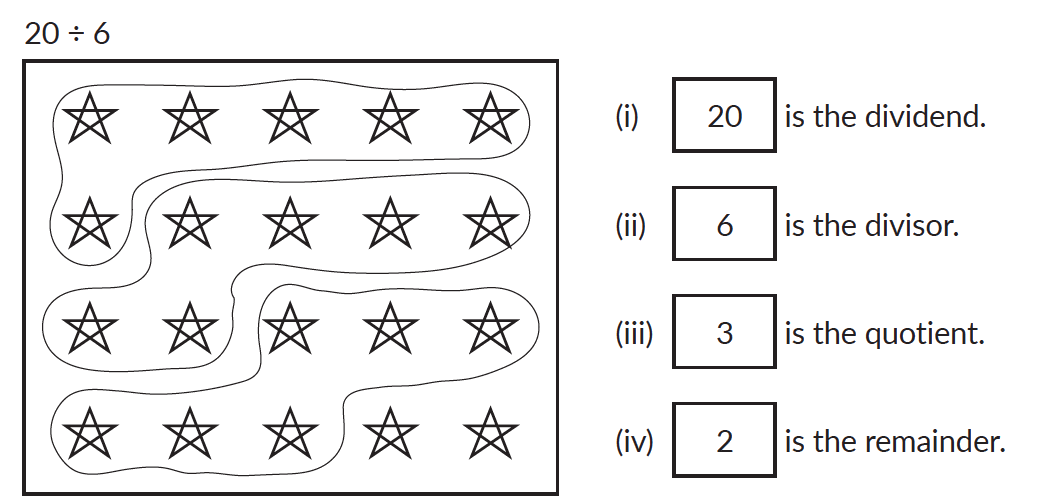## Concept of remainder

The remainder is the amount "left over" or "remaining" after performing division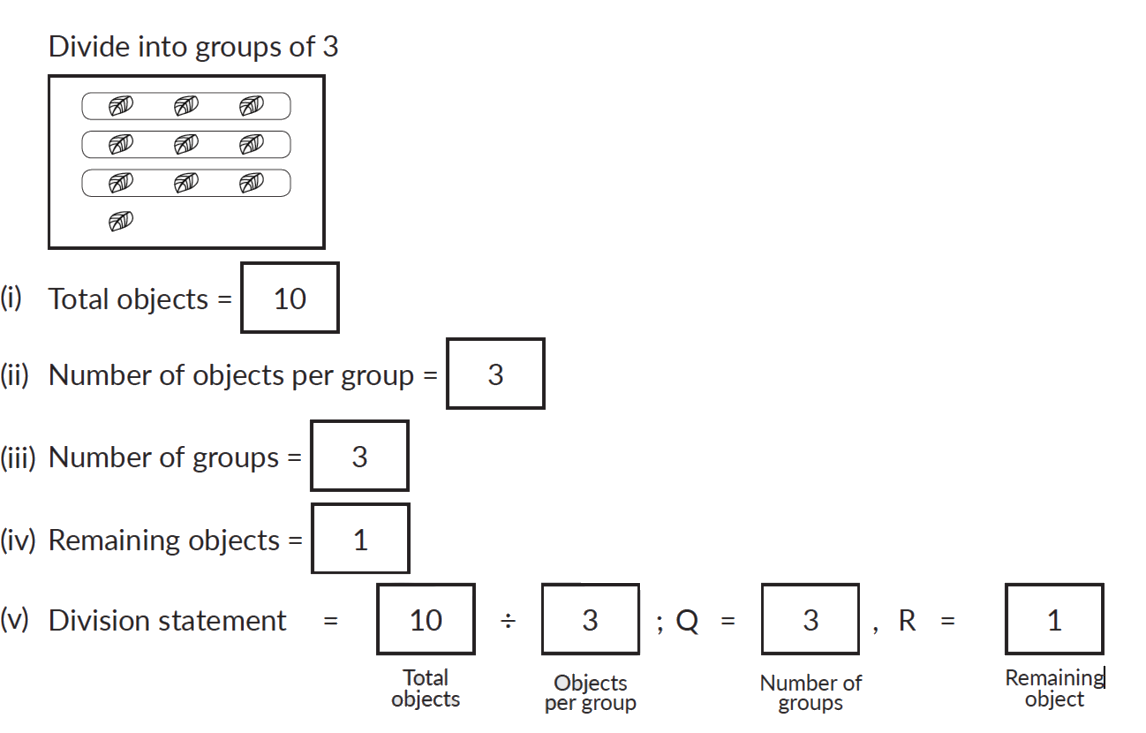## Procedure - Understanding division using the long division method

Division using the long division method can be visualised as shown below.

Step 1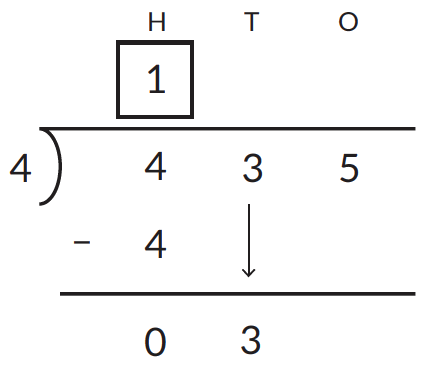Step 2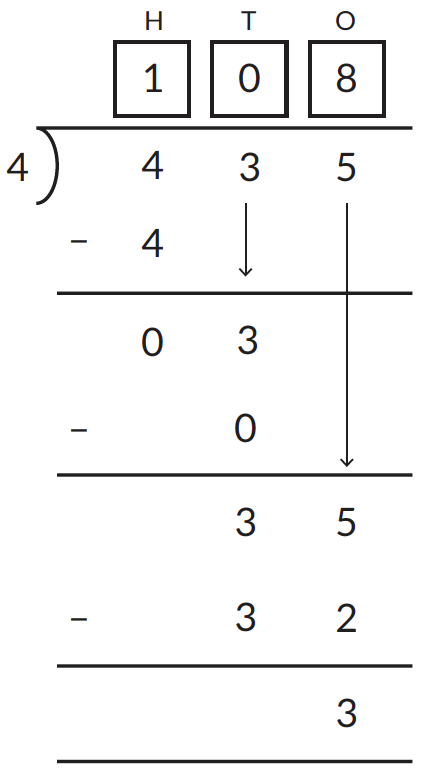## Tips and Tricks

• After solving a division problem, always quickly verify the answer by multiplying the quotient with the divisor and adding the remainder. You should get the dividend.((\text{Quotient}\times \text{Divisor}) + \text{Remainder} = \text{Dividend}\)
• Help children see the connection between a multiplication statement and the division statements that can be derived from it.
E.g. $$3 \times 4 = 12$$ also means $$12 \div 3 = 4$$ and $$12 \div 4 = 3.$$
Using this they can solve many division problems mentally by converting them to a multiplication statement.
• Help children observe that the remainder is always less than the divisor. This fact can help them quickly verify if their working out is correct or incorrect.

### Common mistakes or misconceptions

• Misconception: Students sometimes think that $$12 \div 4 = 4 \div 12.$$ That is, division is commutative
This probably arises because they are extending their knowledge of multiplication to division. Helping them do an activity with counters to show the act of division helps them see the difference between the dividend and divisor.
• In long division, placing zeros in the quotient is often missed. This often happens if students skip a step and pick two digits from the dividend to divide.